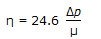# Chemical Engineering - Process Equipment and Plant Design

### Exercise :: Process Equipment and Plant Design - Section 1

11.

In case of a shell and tube heat exchanger, the minimum shell thickness for carbon steel (inclusive of corrosion allowance) depends on shell diameter and is in the range of __________ mm.

 A. 3-5 B. 5-11 C. 8-15 D. 12-18

Answer: Option B

Explanation:

No answer description available for this question. Let us discuss.

12.

The stress developed in a material without any permanent set is called the

 A. ultimate stress B. yield stress C. elastic limit D. breaking stress

Answer: Option C

Explanation:

No answer description available for this question. Let us discuss.

13.

In a shell and tube heat exchanger, the 'tube pitch' is defined as the

 A. O.D. of the tube for square pitch. B. shortest distance between two adj acent tube holes. C. shortest centre to centre distance between adjacent tubes. D. none of these.

Answer: Option C

Explanation:

No answer description available for this question. Let us discuss.

14.

Which of the following is not a valid assumption in continuous binary distillation for calculating the number of equilibrium stages by Mc-Cabe-Thiele's method ?

 A. Sensible heat changes for vapour & liquid are negligibly small. B. Reflux is not a saturated liquid. C. Molar latent heat of the two components are equal. D. Heat of mixing of normal liquid is assumed to be zero.

Answer: Option B

Explanation:

No answer description available for this question. Let us discuss.

15.

Overall distillation column efficiency for most of the refinery columns can be given by (for μ = 0.07 - 1.4 cp and relative volatility < 4) (where η = overall column efficiency, % μ = molal average viscosity of feed at average column temperature and pressure, cp Δp = pressure drop per tray, psi)

 A. η = 17 - 61.1 log μ B.C. η = 1.66 - 0.25 log μ D. η = 0.25 log μ - 1.67

Answer: Option A

Explanation:

No answer description available for this question. Let us discuss.

#### Current Affairs 2021

Interview Questions and Answers